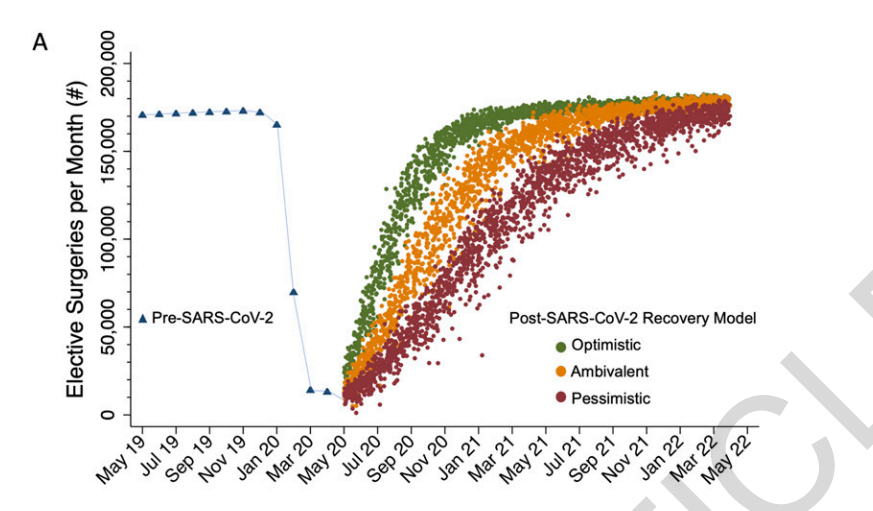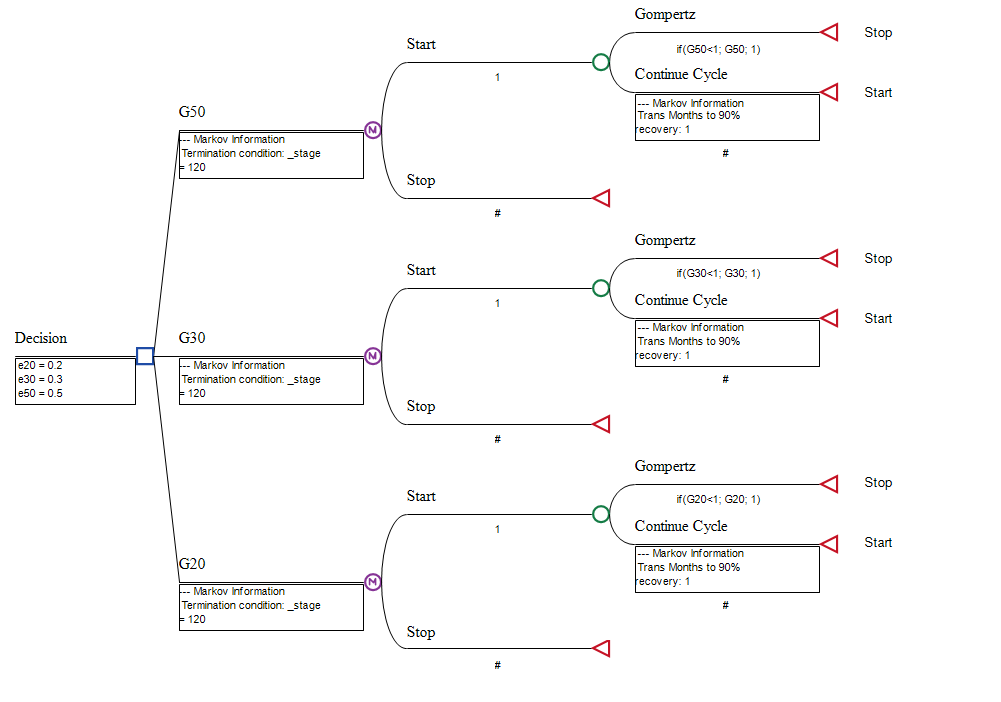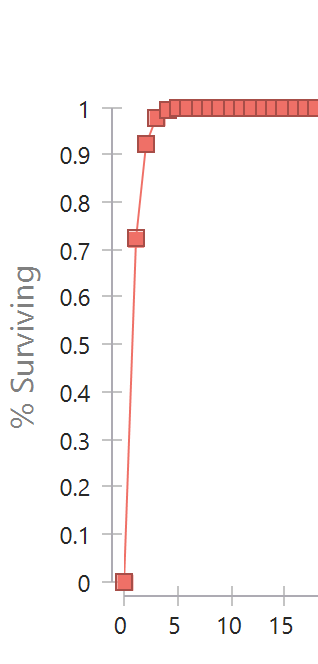# Can I use Treeage to estimate growth with a Gompertz function?

Hi everyone,

I'm trying to figure out whether I can use TreeAge to do this or if I need to use R. There was a recent study that used a Gompertz function to estimate time until a health system has ramped up elective surgeries to pre-COVID levels. They used arbitrary growth values (e.g. 50% which I assume is 50% of the beta value of the Gompertz function) and compared them (please see the attached graph).I built a Markov model in hopes of emulating something like this, but am not sure if I'm on the right track or if this is even possible. This was my model (G50, G30, G20 are modified Gompertz distributions. I had to make sure the returned probability from the function is <1 in order to run the Monte Carlo)This is an example of the output of an individual branch if I do the Markov analysis:I would normalize the Y axis to the total number of cases/surgeries etc. and the bottom will be months. A question is how to get 3 strategies onto one graph with the Monte Carlo data points per the graph above.

Thank you so much!